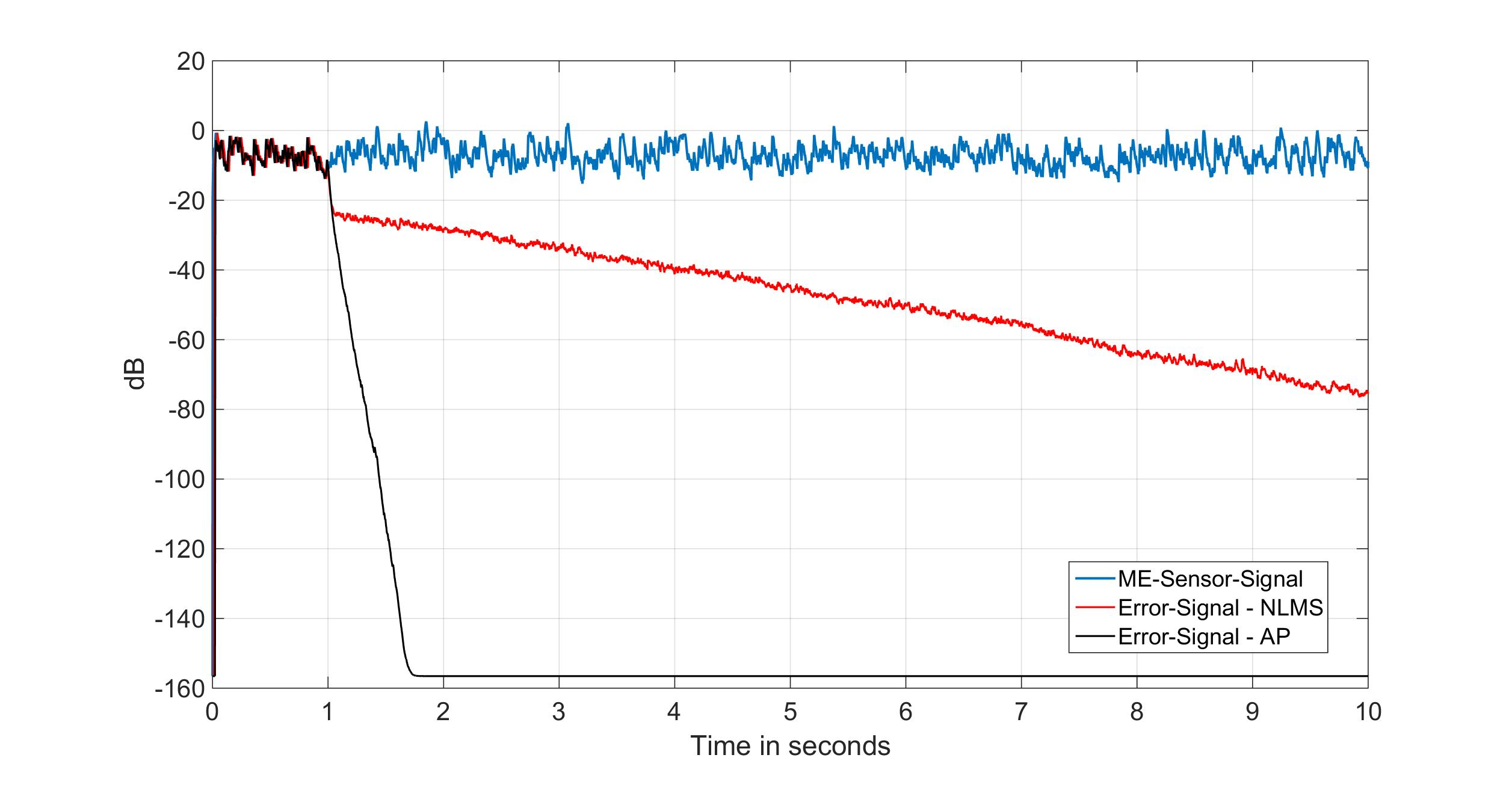## Adaptive Noise Cancellation for Magnetoelectric Sensors### Christin Bald

Project duration: July 2016 - December 2016

The project was supported by the program for promotion of excellent women in science by the Faculty of Engineering / Christian-Albrechts-Universität zu Kiel.

#### Introduction

Within the framework of a collaborative project of different working groups – the SFB 1261 – innovative sensors based on the magnetoelectric effect were designed at Kiel University. Due to their high sensitivity level they have the potential to measure bio magnetic signals with very low amplitudes. In relation to the SQUID (super conducting interference devices) sensors, which were used until now for bio-magnetic measures, the magnetoelectric sensors can work at normal conditions. Unfortunately unmagnetic distortions couple into the sensor as a result of the sensor structure and superpose the magnetic signal .

Thus a noise cancellation based on reference sensors is necessary. Therefore, piezoelectric sensors having the same structure as the magnetoelectric sensors except for the missing magnetostrictive layer are especially suitable .

Different algorithms can be implemented in the adaptive noise cancellation. The most popular algorithm is the NLMS (normalized least mean square) algorithm, which has a very low computational complexity. Unfortunately the algorithm is one-dimensional, so it has no memory and can’t change the direction of the filter coefficients . Thus the NLMS algorithm only works very well in case of white noise. However in reality ambient noise in terms of colored noise exists. Therefore another algorithm than the NLMS algorithm has to be used.

In this project the affine projection (AP) algorithm is used for the noise cancellation of the magnetoelectric sensor. The vector and matrix operations as well as the matrix inversion included in the algorithm, cause a high numerical complexity. This complexity can be reduced by various methods and thus fast affine projection (FAP) algorithms occur .

#### Affine Projection Algorithm

The AP algorithm is a multidimensional algorithm. In contrast to the NLMS algorithm the AP algorithm also takes hypothetical errors into account, which occur when old data vectors are filtered by the new filter coefficients . The AP algorithm is defined as follows:

\begin{eqnarray*} \textbf{e}(n) &=& \textbf{y}(n) - \textbf{X}^{\text{T}}(n)\,\textbf{h}(n-1) \\[1mm] \textbf{u}(n) &=& \Bigl[\textbf{X}^{\text{T}}(n)\, \textbf{X}(n) + \delta \textbf{I} \Bigr]^{-1} \textbf{e}(n) \\[1mm] \textbf{h}(n) &=& \textbf{h}(n-1) + \mu \textbf{X}(n)\, \textbf{u}(n) \end{eqnarray*}

Whereby $$\textbf{X}(n)$$ is the excitation signal matrix and $$\mathbf{h}(n)$$ the adaptive tap weight vector .In the figure below the smoothed power of the magnetoelectric sensor signal and the output signals of the adaptive filters, filtered with the NLMS algorithm and with the AP algorithm, is shown. The AP algorithm converges faster and better than the NLMS algorithm especially in the case of colored noise.

#### Fast Affine Projection Algorithm

The FAP algorithm is a faster version of the AP algorithm in relation to the computational and numerical complexity. To fasten up the AP algorithm the vector and especially the matrix operations should be simplified.

#### Outlook

Various steps to fasten up the AP algorithm are going to be analyzed and implemented for the noise cancellation of the magnetoelectric sensors within this project. These steps are still in progress and will be introduced in the following weeks.

#### References

  J. Reermann et al.: "Adaptive Acoustic Noise Cancellation for Magnetoelectric Sensors," in IEEE Sensors Journal, vol. 15, no. 10, pp. 5804-5812, Oct. 2015.  E. Hänsler and G. Schmidt: "Acoustic Echo and Noise Control – A Practical Approach", Wiley, pp. 95-99, 2004.  S. L. Gay and S. Tavathia: "The Fast Affine Projection Algorithm," Proc. ICASSP, Detroit, MI, USA, pp. 3023-3026, vol. 5, 1995.